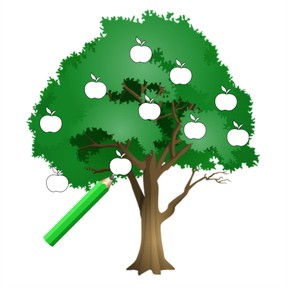Draw quantities 6 to 10

# Draw quantities 6 to 10

Draw quantities 6 to 10

No account needed.8,000 schools use Gynzy92,000 teachers use Gynzy1,600,000 students use Gynzy

## General

Students can draw quantities from 6-10. They know which number belongs to which quantity.

K.CC.B.5

## Relevance

Discuss with students why it is important to be able to draw quantities to 10, namely that they then know which number belongs with which quantity.

## Introduction

Set a maximum of 10 scissors on the table. Ask how many scissors are on the table. Do the same with notebooks. Then show a selection of animals. Where are 8 animals? Ask the students how they know they have the right answer.

## Development

The interactive whiteboard shows a beach with six buckets. Point to the buckets and count them aloud one by one. Every bucket is numbered 1-6. Next show the beach with seven buckets. Point at the buckets and count them aloud. Repeat this until you have reached 10 buckets on the beach. To check that students understand how to draw a quantity, show them a lake which needs 8 boats drawn on it. Ask students if they know how to do this. Draw the boats in the lake. Repeat this, but draw 6 boats. Ask students to individually draw how many balls belong in the goal. Have them hold up their drawings to check their answers. Students then need to say how many eggs are drawn. Ask the students to count aloud.

Check that students can draw the correct number of objects by asking the following questions:
How do you know the number of objects that are drawn?
How can you make sure you draw the right number of objects?

## Guided Practice

Students use a worksheet to practice drawing amounts from 6-10.

## Closing

Discuss that it is useful to know how to draw quantities to 10 because they then know the number that belongs to a quantity. Ask the students to draw a quantity of objects on their paper and then hold it up to check their answers. Next ask a student to come to the front and show them a number card from 6-10. They must draw that amount of dots on the board. The class must answer how many dots they have drawn.

## Teaching Tip

Students who have difficulties drawing quantities can write the numbers 1-10 under the objects they have drawn. This way they have the support of a number line and can immediately see which number is linked to which object.

## Instruction materials

scissors, notebooks, number cards (6-10)

### The online teaching platform for interactive whiteboards and displays in schools

• Save time building lessons

• Manage the classroom more efficiently

• Increase student engagement